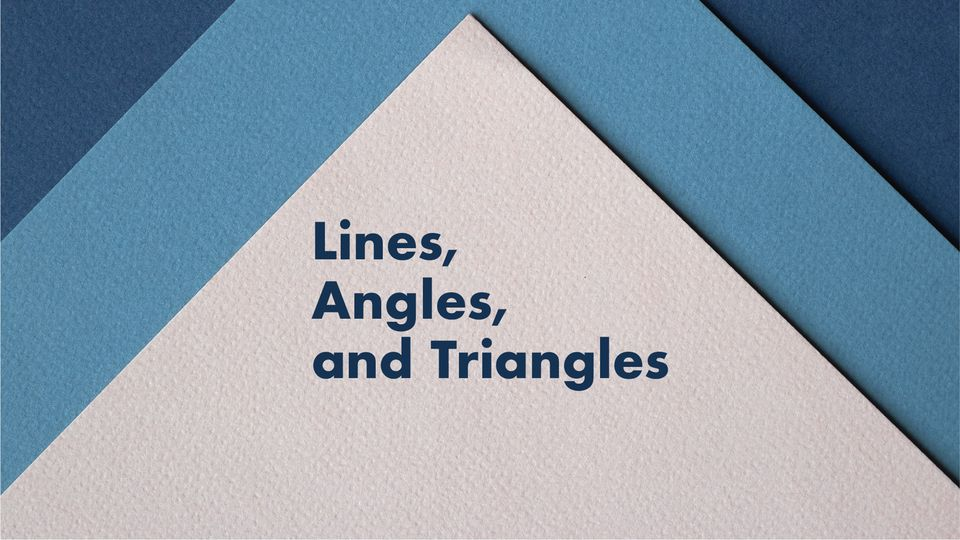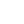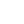Preview the Course
1h 4m

# Lines, Angles, and Triangles

by Karissa Fong | Math10 Lessons 1:04:58
1.1. Trailer 0:31
2.2. Introduction 0:24
3.3. Lines and Angles 8:48
4.4. Triangles 12:28
5.5. Activity 18:58
6.6. Distance Formula 6:07
7.7. Midpoint Formula 4:32
8.8. Pythagorean Theorem 5:16
9.9. Activity 6:43
10.10. Summary & Closing 1:11

1h 4m

14

Students

Learn the foundations of Geometry by starting with defining, identifying, and labeling the various types of lines, angles, and triangles. We will also learn how to use the Distance formula, Midpoint formula, and Pythagorean Theorem to solve real world problems.

Tags:

• Angles
• Geometry
• Graph
• Lines
• Math
• STEM

## Instructor

Hi! I'm Karissa, and I have been a tutor for 4 years with a focus on high school math and science. I am currently studying Environmental Biology at Cal Poly Pomona because I want to find a career that involves conservation efforts. I love to tutor because it allows me to share my passion for learning with others and I hope to inspire others to inquire about the world around them. The world is filled with wonders, so let’s learn about them together!

See full profile